# Syracuse problem

(diff) ← Older revision | Latest revision (diff) | Newer revision → (diff)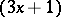-problem, Collatz problem, Hasse algorithm, Hasse–Collatz problem, Kakutani problem, Ulam problem

This problem concerns the iteration of the Collatz mapping that sends a positive integer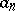to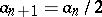(even) or to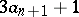(odd). The-conjecture (also called the Collatz conjecture) asserts that for any starting value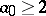there is some iterate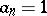.

Some examples are:

a)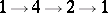;

b)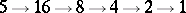;

c)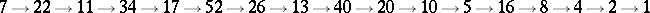.

Ifis allowed to be a negative integer, the conjecture is not true, as is shown by the example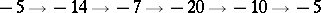. In other words: the Collatz conjecture with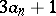replaced by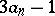does not hold.

This conjecture is generally attributed to L. Collatz, who studied similar problems in the 1930s. It has been numerically verified for all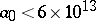[a5]. The conjecture is unsolved (1996) and apparently extremely difficult despite its simple appearance. General references and surveys on the problem are [a3], [a4], [a6].

There is no periodic orbit of the Collatz mapping of period less than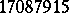, except the orbit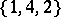. The set of positive integers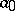that have some iterateless thanhas density one. At leastof all positive integersless thanhave some iterate, [a1].

J.H. Conway [a2] showed that a certain generalization of the-problem is non-computable. He defined a particular mappingfrom the positive integers into the positive integers of the form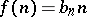, in which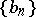is periodic (modulo some) for a fixed modulus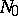, which has the property that the set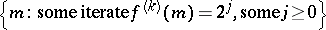is recursively enumerable but not recursive.

How to Cite This Entry:
Syracuse problem. Encyclopedia of Mathematics. URL: http://encyclopediaofmath.org/index.php?title=Syracuse_problem&oldid=11881
This article was adapted from an original article by J.C. Lagarias (originator), which appeared in Encyclopedia of Mathematics - ISBN 1402006098. See original article